Trial ends in

A subscription to JoVE is required to view this content.
You will only be able to see the first 20 seconds.

Reflection and Refraction
Science Education (Physics II)
Series and Parallel Resistors
Science Education (Physics II)
Electric Charge in a Magnetic Field

Reflection and Refraction

Overview

Source: Derek Wilson, Asantha Cooray, PhD, Department of Physics & Astronomy, School of Physical Sciences, University of California, Irvine, CA

Light travels at different speeds depending on the material through which it is propagating. When light travels from one material to another, it will either slow down or speed up. In order to conserve energy and momentum, the light must change the direction in which it propagates. This bending of light is known as refraction. Some fraction of the light is also reflected at the interface between the two materials. In special cases, a light beam can be refracted so sharply at an interface that it is actually completely reflected back into the medium from which it was coming.

Lenses make use of the principle of refraction. Lenses come in two varieties with different curvatures: convex lenses and concave lenses. Convex lenses are often used to focus light but can also be used to create magnified images of objects. When a convex lens causes the light rays coming from an object to diverge, the human eye judges the light to be coming from some point behind the actual object from which the light is originating. The image of the object will in this case be magnified. This type of image is called a virtual image. Concave lenses can also cause light rays to diverge and create virtual images, though the image will be demagnified.

This lab will demonstrate the fundamental law of refraction and will examine the ways in which lenses create images.

Principles

When light hits the interface between two materials, it is bent by some angle that depends on the composition of the two materials. At the boundary, the speed at which the light propagates changes, causing its direction of propagation to change as well. Each medium has a characteristic “index of refraction” that is defined as the ratio between the speed of light in vacuum and the speed of light in the medium: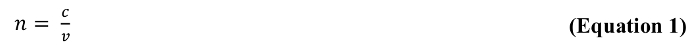where n is the dimensionless index of refraction, c is the speed of light in vacuum in meters per second (m/s), and v is the speed of light in the medium in meters per second (m/s). Light travels more slowly in a medium with a high index of refraction and more quickly in a medium with a low index of refraction.

For a given angle of incidence, өi (angle at which the light hits the boundary between the two media), the angle at which the light beam is refracted, өr, is given by the Law of Refraction, which is more commonly known as Snell’s Law: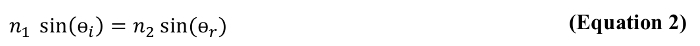where өi and өr are in degrees, and n1 and n2 are the dimensionless indices of refraction of the initial and final materials through which the light is traveling. The refraction angle specifies the direction in which the refracted light wave will travel in the second medium (see Figure 1). Some fraction of the incident light is also reflected back into the first medium at an angle equal to the angle of incidence.

An interesting phenomenon occurs when light goes from a material with a high index of refraction to one with a lower index. There is a critical incident angle at which the refracted angle will become 90°. If light hits the boundary at the critical angle, the refracted beam will travel along the boundary between the media, and some light will be reflected back into the high index of refraction material (see Figure 2). If light hits the boundary at an angle greater than this critical angle, it will be completely reflected back into the high index of refraction material in an event called total internal reflection.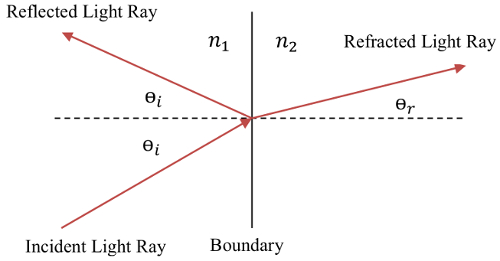Figure 1: An incident light ray on the boundary between two media results in a reflected ray and a refracted ray.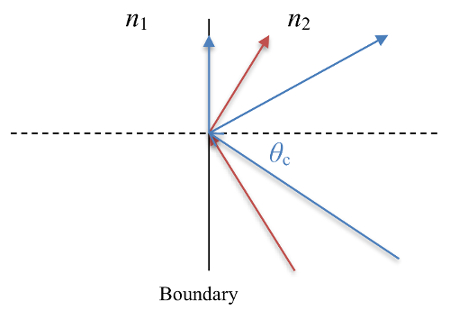Figure 2: Total internal reflection when n2 > n1. The blue ray is incident at the critical angle and results in a refracted ray traveling along the interface and a reflected ray. The red ray is incident at an angle larger than the critical angle and leads to a totally internally reflected ray.

Lenses take advantage of refraction to create real and virtual images of objects. A real image is an image formed by the physical convergence of light rays that came from an object. A virtual image is formed when light rays appear to converge but do not actually physically converge. Our eyes construct a point of origin for diverging rays, and this point of origin serves as the source of the virtual images even though the light rays do not actually converge at this point. Examples of real and virtual images formed by a convex lens are shown in Figure 3. Lenses have a characteristic length called the “focal length”, which is the distance from the lens at which the light rays originating infinitely far away will be focused after passing through the lens. Given the focal length of a lens, the distance between the object and the lens will determine the location of the image according to the thin lens equation: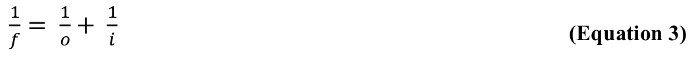Where f is the focal length of the lens in meters (m), o is the distance between the lens and object in meters (m), and i is the distance between the lens and image in meters (m). If the object distance is taken to be a positive quantity, then, if the image distance is positive, the image will be real and will be located on the side of the lens opposite to the object. If the image distance is negative, the image will be virtual, magnified, and located on the same side of the lens as the object.Figure 3: A convex lens producing real and virtual images. Real images form from the true convergence of light rays. Virtual images are constructed by our eyes from diverging light rays.

Procedure

1. Determine the index of refraction of water using Snell’s Law (Law of Refraction) and find the critical angle for total internal reflection.

1. Obtain a specialized refraction tank with a light source.
2. Fill the refraction tank with water and turn on the light source. Direct the beam from the light source into the half of the tank filled with water. It may be necessary to dim the lights in the room.
3. Use the protractor on the refraction tank to measure the beam’s angle of incidence (the angle measured in the half of the tank filled with water) and angle of refraction (the angle measured in the half of the tank filled with air) for the water-air interface in the tank.
4. Use the measured angles and the index of refraction for air (nair = 1.00) to calculate the index of refraction for water.
5. Repeat the previous steps for a few incident angles varying from 0° to just under 90°.
6. As the incident angle is increased, one will notice that the refracted light beam can no longer be seen in the half of the container containing air. Slowly rotate the light source about the tank until the point at which the light beam first disappears from the air is reached. This is the critical angle for total internal reflection.
7. If the light source is further rotated, it should be observed that the beam reflects back into the water.
8. Move the light source so that the beam enters the half of the tank filled with air first before traveling into the water. Record a few angles of incidence and refraction under this condition. Note that, previously, the angle of incidence was the angle at which the light traveled through the water. Since the light is now going through the air first, the new angle of incidence is the angle at which it travels through the air, and the new angle of refraction is the angle at which it travels through the water.
9. Observe that total internal reflection does not occur in this configuration. Total internal reflection only occurs when light goes from a medium with a high index of refraction to a medium with a lower index of refraction.

2. Measure the focal length of a lens and create real and virtual images of an object.

1. Obtain a convex lens, a concave lens, a sheet of white paper, a ruler, and a small distinctive object. It also helps to have an optical bench with holders for the lenses and object as well as an apparatus to hold the sheet of paper vertically.
2. Place the convex lens between the object and the piece of paper, all in a line and at the same height.
3. Move the object and paper around until a sharp image of the object appears on the paper. The image on the paper is a real image.
4. Measure the distance from the lens to the object and the distance from the lens to the paper. Use the thin lens equation to determine the focal length of the lens.
5. Place the paper aside and move the object closer to the lens until the distance between the lens and object is less than the focal length of the lens.
6. Look through the lens: a magnified version of the object should be seen. This image is a virtual image.
7. Replace the convex lens with the concave lens. Look through the concave lens: now a demagnified version of the object is visible. This is also a virtual image.

Light reflects and travels at different speeds and direction, or refracts, depending on the material through which it is propagating, causing many interesting optical phenomena.

When a ray of light strikes the surface of a glass block, a part of it changes direction at the interface to return into the medium from which it originated; this is reflection. And the rest of the light changes its direction at the interface and travels through the block of glass to conserve energy and momentum; this is refraction.

Lenses found in optical systems like microscopes make use of reflection and refraction to create images that can be perceived by the human eye.

Here, we will first discuss the principles and parameters of reflection and refraction. Then we will demonstrate these phenomena in a system where air and water are the two media. Next, we will study the ways in which lenses create images, followed by a few applications in the field of optics.

To understand the principles and parameters of reflection and refraction, let us pick two media - water and air.

The first key parameter to note is "refractive index", 'n' - a characteristic of the medium through which light travels. It is defined as the ratio between the speed of light in vacuum, 'c', to the speed of light in the medium, 'v'. As the n of air is lower than water, light travels more slowly through water as compared to air.

Let us now assume that the two media, water and air, are in contact with each other along an interface.

Now when light travels from water to air and hits the interface, some of it is reflected at the interface, and the remaining is refracted or bent by an angle that depends on the refractive indices of the two media. Both reflection and refraction are also dependent on another parameter - angle of incidence, or θi.

This is the angle between the incident light and the normal to the air-water interface inside the first medium, water. The 'angle of reflection' is measured between the reflected light and the same normal inside the first medium, water, and is equal to the angle of incidence. Whereas the 'angle of refraction', or θr is the angle between the refracted light and the normal to the air-water interface in the second medium, air.

The angle of refraction is thus dependent on the angle of incidence and the refractive indices of the two media. The law of refraction or Snell's Law provides a relationship between all of these parameters.

Now, if the angle of incidence is slowly increased, at one point the light would appear along the water-air interface, and the angle of refraction will equal 90 degrees. This incident angle is called the 'critical angle'. Note that it can only happen if the refractive index of the first medium is greater than the second one.

Under this same condition, if the angle of incidence is increased further, then the light beam is refracted so sharply that it is actually completely reflected back into the first medium from which the light originated. This phenomenon is called Total Internal Reflection.

Having reviewed the parameters that affect reflection and refraction, let's see how to perform an experiment in a physics lab that validates these principles. Gather all the necessary materials and equipment including a specialized refraction tank with a light beam.

Fill one half of the refraction tank with water. Turn ON the light beam and direct the beam into half of the tank filled with water.

Using a protractor, measure the light beam's angle of incidence or the angle measured in the water between the light beam and the normal to the air-water interface. Also, measure the angle of refraction or the angle measured in air between the light beam and the normal to the air-water interface

Now, as the incidence angle is increased, a point is reached at which the light beam appears along the air-water interface. Make a note of this incidence angle, as it is the critical angle for total internal reflection.

Next, continue to increase the angle of incidence by rotating the light source counter-clockwise. The refracted beam now gets completely reflected into the water demonstrating Total Internal Reflection.

Subsequently, move the light source so that the beam enters the air-half of the tank first before travelling into the water. Repeat the protocol for the new light beam path for various angles of incidence and record the corresponding angle of refraction.

Now let's talk about lenses, which take advantage of reflection and refraction of light to create real and virtual images of objects. All lenses, whether convex or concave, have a focal length 'f', which is the distance from the lens at which light rays originating from infinitely far away will be focused after passing through the lens. For convex lenses f is positive and for concave lenses f is negative.

When an object is placed in front of a lens, it creates an image. The 'Thin Lens Equation', provides a mathematical relationship between the focal length 'f', the distance between the object and the lens, 'o', and the distance between the lens and the image, 'i'.

It is this mathematical image distance 'i' that tells us whether an image formed by the lens is real or virtual. If the mathematically calculated 'i' is positive then the image formed will be real, and if it is negative the image will be virtual.

For a convex lens, when the object distance 'o' is greater than the focal length 'f', the mathematically calculated image distance 'i' will be positive and a real image is formed. This is due to the physical convergence of light rays that come from the object, like the image captured by a camera or a microscope.

However, when the object distance 'o' is less than the focal length 'f', the mathematically calculated image distance 'i' is negative and a virtual image is formed. This is because the light rays appear to converge but actually physically diverge, and our eyes construct a point of origin for them. This is observed in the case of a magnifying glass, where a magnified virtual image is formed.

For concave lenses, the light rays that come from the object pass through the lens and always diverge. Thus, the calculated 'i' is always negative and the image created is always virtual.

In this section, we will validate the formation of real and virtual images using simple convex and concave lenses. Gather the required materials, namely a convex lens, a concave lens, a sheet of white paper, a small distinctive object, and a clamp to hold the paper vertically

First, place the convex lens between the object and the piece of paper. Make sure that they are all in line and at the same height.

Move the object and paper around until a sharp image of the object appears on the paper. This image seen on the paper is a real image, as it can be captured on a screen.

Now measure the distance from the lens to the object and from the lens to the paper. Use the thin lens equation to determine the focal length of the lens.

Next, place the paper aside and move the object closer to the lens until the distance between the lens and the object is less than the focal length of the lens. Look through the lens and observe the image.

Replace the convex lens with a concave lens. Look through the concave lens and observe the demagnified virtual image.

Now that we have completed the experimental protocol, let's review how to analyze the data obtained. In the first experiment, we measured the angle of incidence and the angle of refraction at the water-air interface.

By using the Snell's law and substituting the values for these angles into the equation, along with the refractive index of air, we can calculate the refractive index of water, which comes out to be 1.33.

This calculation can then be repeated for the various incident and refraction angles. The average of all the calculated refractive indices will provide a more accurate measurement of the index of refraction of water.

We can also calculate the critical angle for total internal reflection using Snell's law. This is the incidence angle when the refraction angle equals 90 degrees. Rearrange this equation to solve for critical angle.

Using the previously calculated average for the refractive index of water, Snell's law predicts that the critical angle of incidence is 48.8 degrees. This is very close to the angle measured experimentally, thus verifying Snell's law.

When the light beam is projected from air to water, total internal reflection does not occur even at angles greater than 48.8 degrees as light is now traveling from a medium of lower index to higher.

In the experiment with the lenses, the thin lens equation reveals that for an object distance of 11.02 centimeters from the lens and an image distance of about 9.21 centimeters, the focal length of the lens is about 5.02 centimeters.

In the case where the object is observed through a convex lens, at a distance less than its focal length, a magnified version of the object is observed. This is a virtual image, as this image cannot be captured on a screen. Similarly, when using the concave lens, a demagnified virtual image of the object is observed.

Optics, specifically optical lenses, is used in every walk of life from photography to medical imaging to the human eye.

Optical fibers are used for data transmission in many current day applications, like transmission of telephone signals. These fibers consist of a core, cladding, and a protective outer coating or buffer, and other strengthening layers.

The cladding guides the data in the form of light pulses along the core using the method of total internal reflection. This property of data transmission enables fiber optic cameras used by doctors to view confined spaces in human body.

Microscopy is the field of using microscopes to view objects that are not visible to the naked eye. Optical or light microscopy involves passing visible light, which is refracted through or reflected from the sample, through a single or multiple lenses to allow a magnified view of the sample. The resulting image can be detected directly by the eye, or captured digitally.

You've just watched JoVE's introduction to reflection and refraction. You should now understand the principles of refraction, Snell's law, and total internal reflection and also the theory behind lenses and how they create images. As always, thanks for watching!

Results

Snell’s Law dictates the angle at which light will be bent when crossing the boundary between two media. The measured incident and refracted angles at the water-air interface are given in Table 1. Below, a sample calculation giving the index of refraction for water using Snell’s Law is shown for an angle of incidence equal to 30.1° as the light goes from the water to air: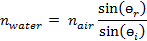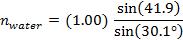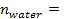1.33

The calculation can be repeated for the various angles in Table 1, and the average of the measurements will provide an even better measurement of the index of refraction than any of the individual measurements will provide.

Table 1: Results.

 Interface өi өr nwater Water → Air 10.0 13.5 1.34 Water → Air 19.8 26.6 1.32 Water → Air 30.1 41.9 1.33 Air → Water 20.1 15.1 1.32 Air → Water 44.9 32.0 1.33 Air → Water 75.2 46.7 1.33

The critical angle for total internal reflection occurs when the refraction angle is equal to 90°. For the water-air interface, Snell’s Law predicts that the critical angle of incidence is 48.8°.

It is worthwhile to note that the refracted beam could still be observed at an angle greater than 48.8° when looking at the interface at which the light beam went from air into the water. It is only at the boundary at which the beam went from the water to the air that the beam was internally reflected at angles greater than 48.8°. Total internal reflection can only occur when light goes from a medium with a high index of refraction to a medium with a lower index of refraction.

For the lens part of the experiment, when the object was placed at about o = 11.02 cm, the image came into focus at about 9.21 cm. The thin lens equation then reveals the focal length of the convex lens to be about 5.02 cm.

Applications and SummaryX